## Topic 16.1: Simplex Method (Maple)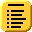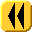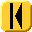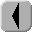Introduction Notes Theory HOWTO Examples Engineering Error Questions Matlab Maple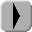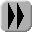The simplex algorithm is implemented in the simplex package of Maple.

constraints := {3*x + y + z <= 4, 2*x + 2*y + z <= 5, x + 3*y + z <= 4, x + y + 2*z <= 5}; definiteness := {x >= 0, y >= 0, z >= 0}; simplex[maximize]( 3*x + 4*y + 2*z, constraints union definiteness );
```
For help on this package or on the maximize routine, enter

?simplex
?simplex,maximize